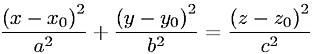Equations > Geometry > Curves and Shapes > Equation of an Elliptic Cone

Equation of an Elliptic ConeLatex Code:

MathML Code:

 $\frac{\left(x-{x}_{0}\right)}^{2}{a}^{2}+\frac{\left(y-{y}_{0}\right)}^{2}{b}^{2}=\frac{\left(z-{z}_{0}\right)}^{2}{c}^{2}$

MathType 5.0: